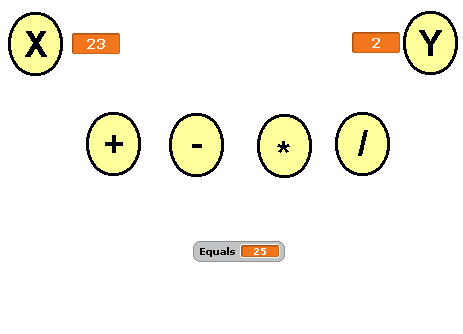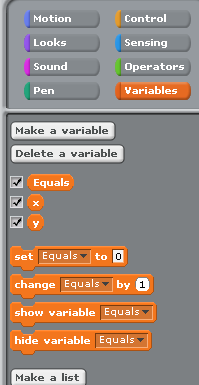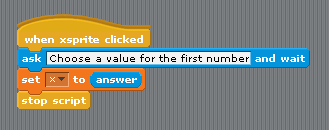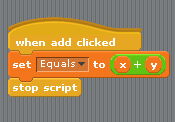# Scratch ProgrammingSimple Calculator

## Program 2 - Simple Calculator

### Introduction

This second program is based on the same programming concepts that you met in the story program. The difference is that we will be working with variables that store numbers instead of words or strings.

The program will look something like this,X and Y are the two numbers we want to use in our calculation. To enter the values for these variables, you need to click on the sprite with the X or Y on it.

If you click on the plus, X and Y are added together and shown next to where it says equals.

This is the start of a calculator program, you will be able to add more features later.

To start you off, you can download a version of the program where the sprites have already been created and named for you. Right mouse click on the file and choose Save Target As or the equivalent for your browser.

simple calculator - no scripts.sb

### Starting Off - Variables

This program works with 3 variables,

• x - to store the first number entered
• y - to store the second number entered
• Equals - to store the result of any calculations

When you make these variables, they need to be For All Sprites.

You want these variables to be shown on the screen. So you make sure the box to the left of the variable name is checked like you see in the following image,One last job is to change the way that X and Y are displayed. Right mouse click on the variable name on the stage and choose Large Readout.

### Getting Input From The User

The following script can be added to the xsprite scripts section. The first block tells the program that these blocks are executed when the user clicks on the sprite. You should recognise the other blocks from the story program.You will need to create a similar script for the ysprite. When you have completed both scripts, you should test that your program allows you to change the values stored in the X and Y variables when you click on the relevant sprite.

### Performing A Calculation

Calculations are performed when the user clicks on one of the + - * / sprites. Below is the script that adds together X and Y and stores the result in the variable called equals.Add this script and test that it works as it should.

You will need to create an appropriate script for each of the remaining sprites. When you have finished, you should have a basic calculator.

The immediate thing you can do to extend your program is to add more operations. You will need to make an appropriate sprite for each one. You can do this by duplicating one of the existing sprites, going to costumes and editing the costume. Click the T for text tool and you can edit the text on the sprite. The following calculations might be useful,

• X2 - X squared or X * X
• X3 - X cubed or X * X * X
• Round Result - Round the value stored in equals to the nearest whole number
• X mod Y - The remainder when X is divided by Y
• 2 * PI * X - The circumference of a circle with a radius of X
• PI * X2 - The area of a circle with a radius of X
• √X - The square root of X.

Other functions that would help would be,

• AC - A button that sets all of the variables to 0 - clears the calculator
• Copy - A button that copies the value stored in Equals and stores it in X so that the answer to a calculation can be used in the next one.
• M, M+, MR - Write to memory, add to memory, recall memory. These buttons would allow you to store numbers and use them in later calculations.

### For The Explorer

Try to make the calculator look and work like a normal calculator. This is a bit fiddly but it can be done in Scratch.